# 常见神经网络

Posted by MaggicQ on October 13, 2018

# 常见神经网络

## 卷积神经网络• 式中$z_{i,j,k}$是第$l$层卷积层中的位于第$k$个特征层（feature map 或者说 channel）中的第$i$行第$j$列的神经元的输出。
• $s_h$以及$s_w$分别对应高度$H$以及宽度$W$方向上的步伐（strides），$f_h$以及$f_w$对应的是一次卷积操作范围的高度和宽度（比如上面的动图就是$3 \times 3$ ），$f_{n’}$表示前一层即第$l-1$层的特征层的数量。
• $x_{i’, j’, k’}$是第$l-1$层上的位于第$k’$个特征层中的第$i’$行第$j’$列的神经元的输出。
• $b_k$是第$l$层卷积层的偏差（bias）
• $w_{u,v, k’, k}$ 是第$l$层卷积层中的第$k$个特征层上的神经元与前一层卷积层中位于第$u$行第$v$列第$k’$个特征层的神经元的输出的权重连接。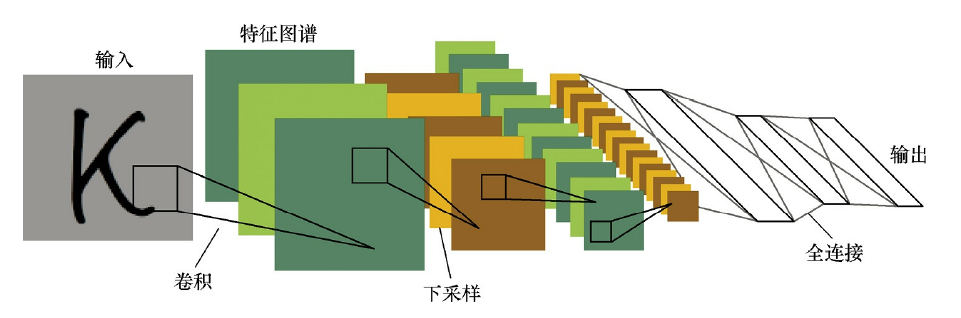### 卷积操作的特性

1. 稀疏交互。在卷积神经网络中，卷积核尺度远小于输入的维度，这样每个输出神经元仅与前一层特定局部区域内的神经元存在连接权重(即产生交互，如下图所示)，我们称这种特性为稀疏交互。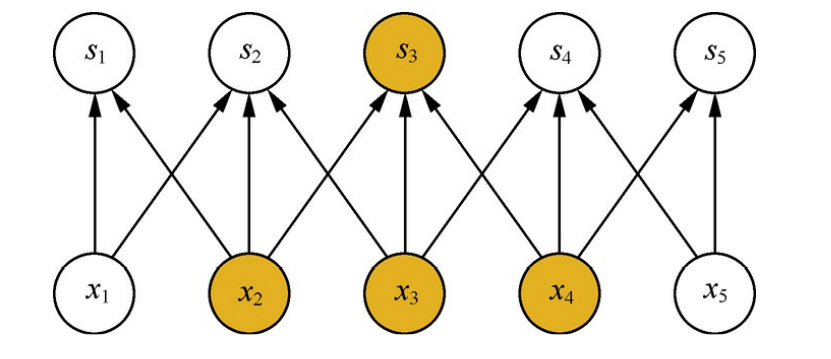稀疏交互的物理意义是，通常图像、文本、语音等现实世界中的数据都具有局部的特征结构，我们可以先学习局部的特征，再将局部的特征组合起来形成更复杂和抽象的特征。

2. 参数共享。在卷积神经网络中，卷积核中的每一个元素将作用于每一次局部输入的特定位置上。根据参数共享的思想，我们只需要学习一组参数集合，而不需要针对每个位置的每个参数都进行优化，从而大大降低了模型的存储需求。

参数共享的物理意义是使得卷积层具有平移等变性。假如图像中有一只猫，那么无论它出现在图像中的任何位置，我们都应该将它识别为猫，也就是说神经网络的输出对于平移变换来说应当是等变的。

### 池化操作

• 均值池化通过对邻域内特征数值求平均来实现，能够抑制由于邻域大小受限造成估计值方差增大的现象，特点是对背景的保留效果更好。
• 最大池化则通过取邻域内特征的最大值来实现，能够抑制网络参数误差造成估计均值偏移的现象，特点是更好地提取纹理信息。

### 用于文本分类的CNN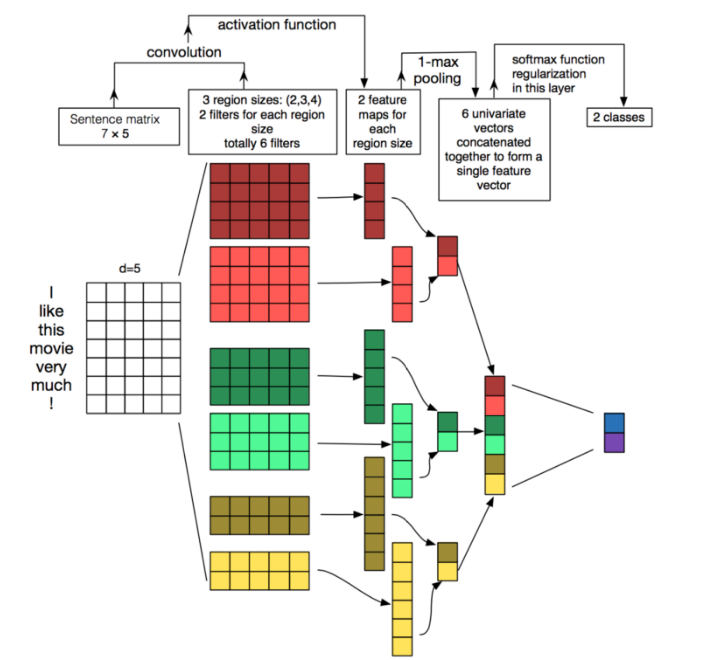## 深度残差网络

ResNet的调整如下图所示：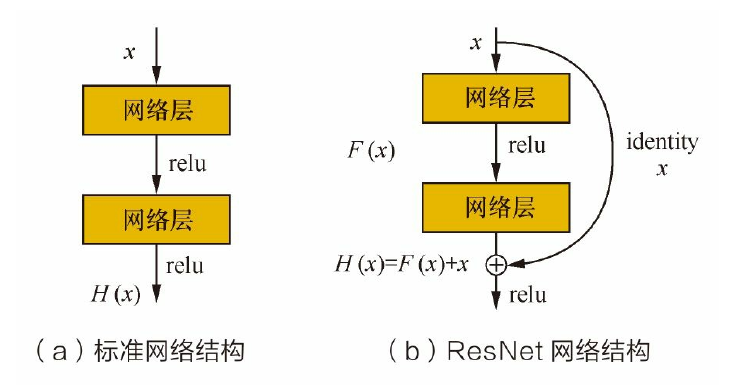ResNet可以有效改善深层的神经网络学习问题，使得训练更深的网络成为可能。

## 循环神经网络(Recurrent Neural Network,RNN)

### Vanilla RNN### LSTM

LSTM的结构如下所示：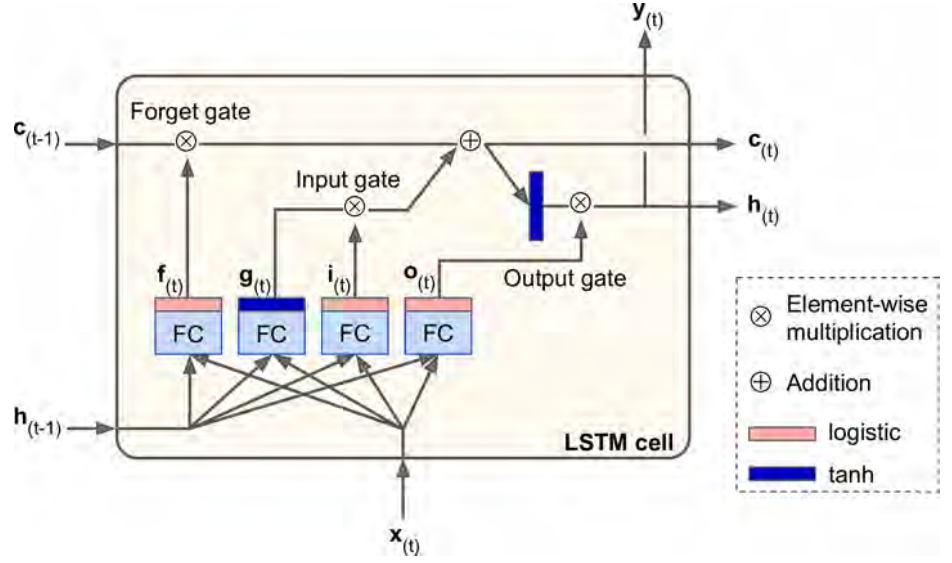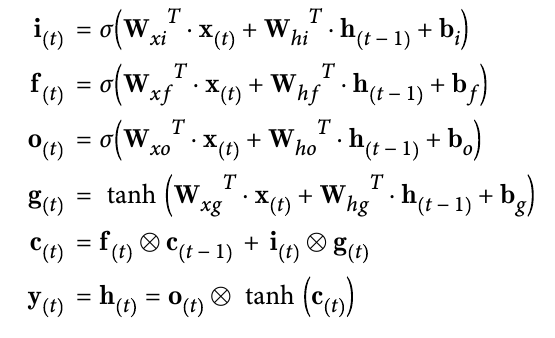LSTM比起Vanilla RNN的优势

LSTM能学习到序列之间的长距离依赖。而Vanilla RNN中由于梯度被近距离梯度主导，远距离的梯度很弱（或者消失），导致模型难以学到远距离的依赖关系。

### GRU

GRU是对LSTM单元的一种简化，GRU的结构如下所示：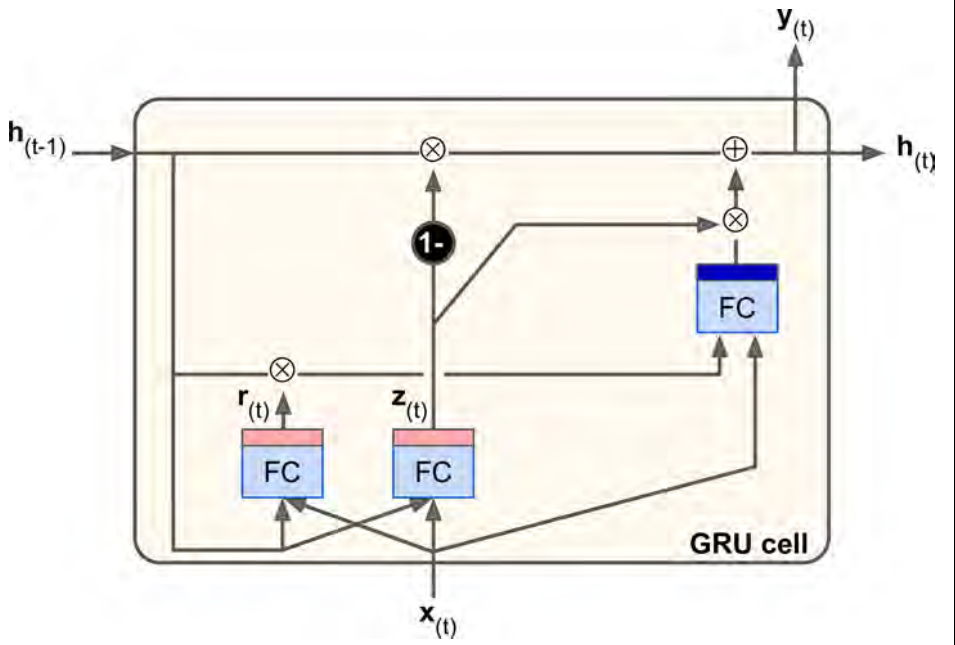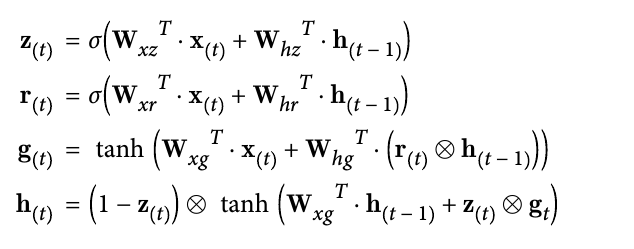• LSTM中有两个状态向量$h_t, c_t$， GRU只有$h_t$
• 移除了输出门，$y_{(t)} = h_{(t)}$
• GRU中的遗忘门以及输入门是联动的。如果遗忘门为1，那么输入门就为0，反之亦然。

### RNN的应用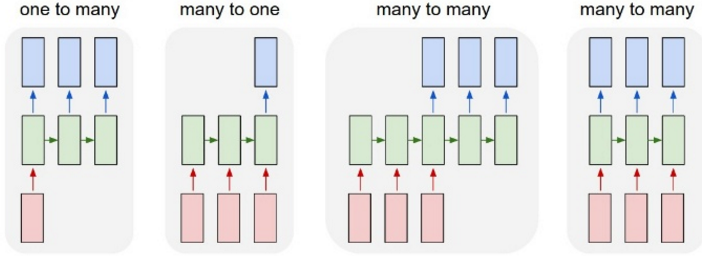• 一对多，比如图像的标题生成

• 多对一，比如句子的情感分析
• 多对多（seq2seq模型）：
• encoder-decoder模式，RNN在处理整个输入序列之后再产生另外一个序列，如文本翻译或者文本摘要，encoder-decoder模型有助于RNN获得全局的信息。
• 无时差的序列到序列转化，比如给一个录像中的每一帧贴标签。

## 参考

A technical report on convolution arithmetic in the context of deep learning

LSTM如何来避免梯度弥散和梯度爆炸？

The unreasonable effective RNN